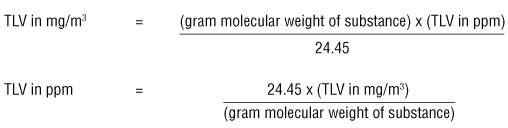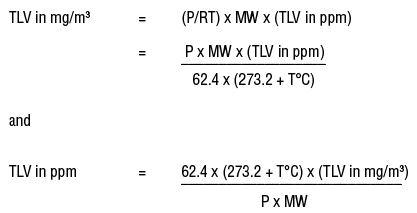# Converting Occupational Exposure Limits from mg/m3 to ppm

## When can I convert mg/m3 to ppm?

Occupational exposure limits (OELs, threshold limit values (TLVs), permissible exposure levels (PELs), etc.) can be expressed in parts per million (ppm) only if the substance exists as a gas or vapour at normal room temperature and pressure. This state is the reason exposure limits are usually expressed in mg/m3.  Some OELs may be expressed in units such as fibres/cc (e.g., for asbestos). OELs for metals, salts, and other compounds that do not form vapours at room temperature and pressure are expressed in mg/m3 only.

## What is a common way of converting mg/m3 to ppm?

The ACGIH booklet "Threshold Limit Values (TLVs™) for Chemical Substances and Physical Agents and Biological Exposure Indices (BEIs™)" provides the following conversion formulas:Where 24.45 is the volume in litres occupied by a mole of air at 25ºC and 760 torr.

These formulas can be used when measurements are taken at 25°C and the air pressure is 760 torr (= 1 atmosphere or 760 mm Hg).

## What is gram molecular weight?

Gram molecular weight is the molecular weight (MW) of a substance expressed in grams. For example, the gram molecular weight for toluene is 92.13 g. since the molecular weight is 92.13. A gram molecular weight is also called a gram mole.

## How do I convert mg/m3 to ppm at different temperatures and pressures?

The number 24.45 in the equations above is the volume (liters) of a mole (gram molecular weight) of a gas or vapour when the pressure is at 1 atmosphere (760 torr or 760 mm Hg) and at 25°C.

To convert mg/m3 to ppm at other temperatures and pressures, one must calculate the volume of one gram molecular weight of  the airborne contaminant (e.g., 92.13 grams of toluene) at that temperature and pressure by using the ideal gas law formula:

PV = nRT

where n is the number of moles, R is the ideal gas constant (62.4 L torr/mol K when the temperature (T) is in kelvins (K)),  T is the temperature in Kelvins (273.16 + T°C); and P is the pressure in mmHg.

The gas constant can have different values if the temperature and pressure are expressed in different units from those in the above equation (e.g., R= 0.082 4 l . atm / mol . K )

For n = 1
V = RT/ P

V can be substituted in the formulas for converting between mg/m3 and ppm.• Fact sheet last revised: 2022-07-14